# Prime factors

We know Prime =  Prime numbers    2, 3, 5, 7, 11, 13.............

We know Factors =  Take a number apart using ladders

So logically Prime Factors are:Example 1

What is the prime factorization of 12?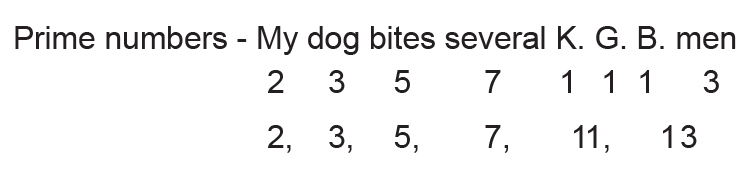Factor the number 12

Write down the number and put two ladders up against it.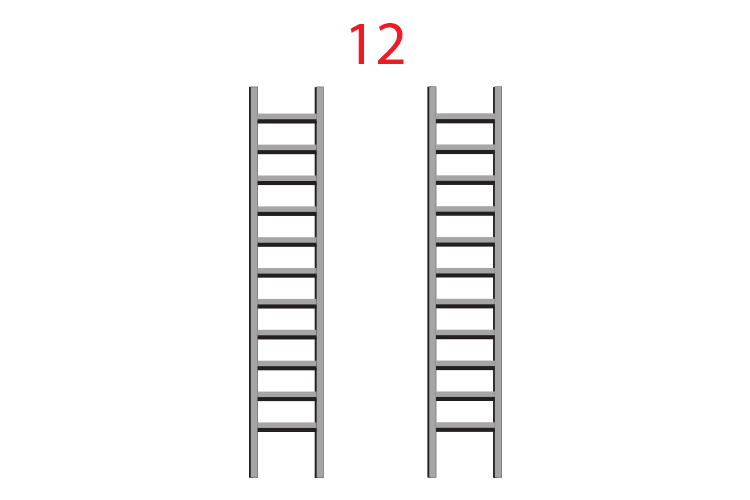Then write the numbers from 1 down the left ladder.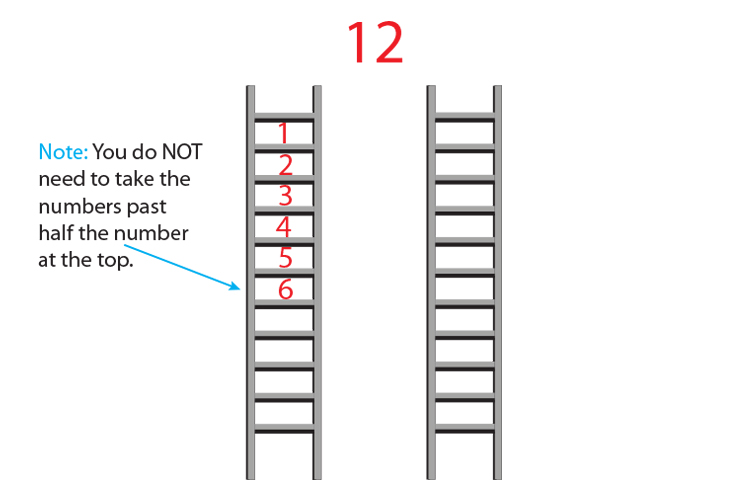Now fill the right ladder with the numbers that multiply to give 12.Therefore the factors of 12 are:

1 and 12

2 and 6

3 and 4

Alternatively the factors of 12 are 1, 2, 3, 4, 6 and 12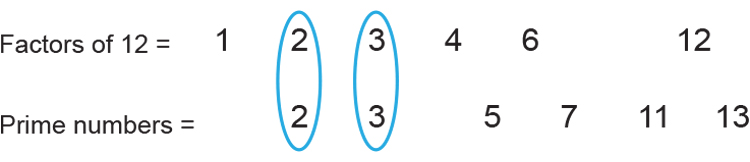The only prime numbers that are also factors of 12 are:

2 and 3

So we have to use only these two numbers to multiply to 12.

Try 2times3=6

Try (2times3=6)times2=12

So the answer is 2times3times2

or 2times2times3

or 2^2times3

Example 2

What are the prime factors of 24?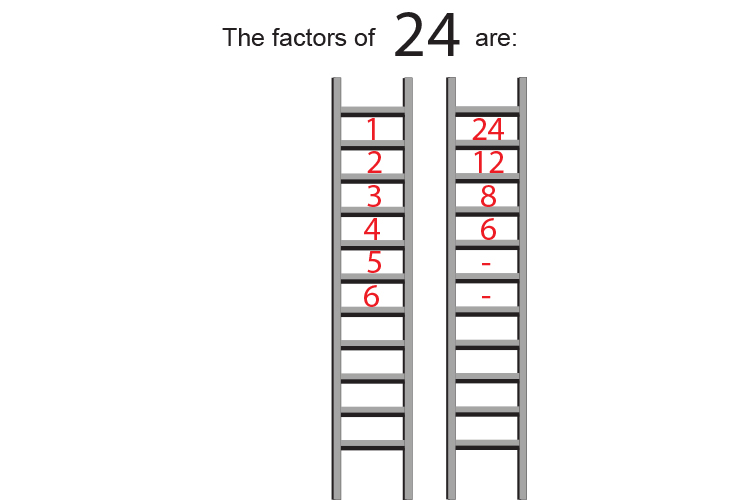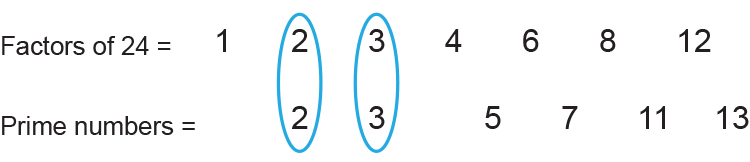The only prime numbers that are also factors of 24 are:

2    and    3

So we have to use only these two numbers to multiply to 24.

Try 2times3=6

Try (2times3=6)times3=18  - can't multiply to get 24

Try (2times3=6)times2=12

Try (2times3times2=12)times2=24

So the answer is 2times3times2times2

which is 2times2times2times3

or 2^3times3

Example 3

What is the prime factorisation of 70?Factor 70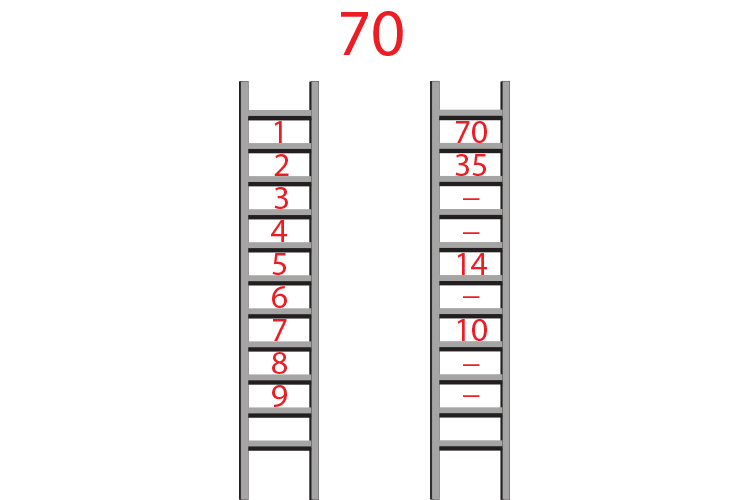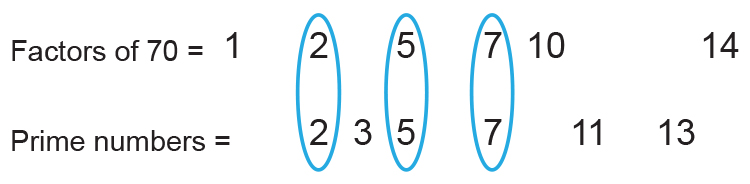The only prime numbers that are also factors of 70 are:

2, 5 and 7

So we have to use only these 3 numbers to multiply to 70

Try 2times5=10

Try (2times5=10)times7=70

So the answer is 2times5times7

Example 4

What is the prime factorisation of 147?Factor 147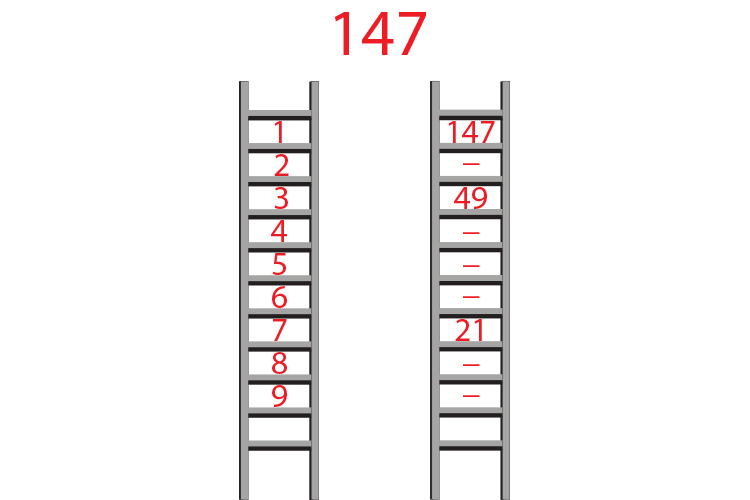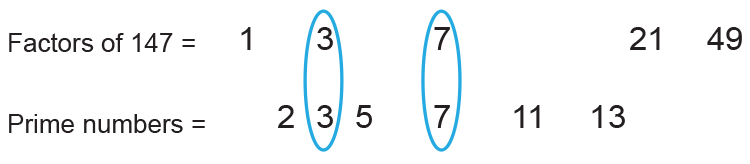NOTE:

21 and 49 are not prime numbers.

The only prime numbers that are also factors of 147 are:

3 and 7

So we have to use only these two numbers to multiply to 147.

Try 3times7=21

Try (3times7=21)times7=147

So the answer is 3times7times7

or  3times7^2

Example 5

What is the prime factorisation of 17?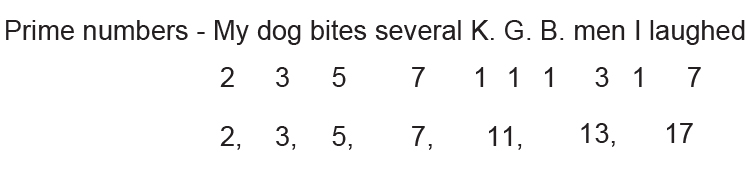Factor 17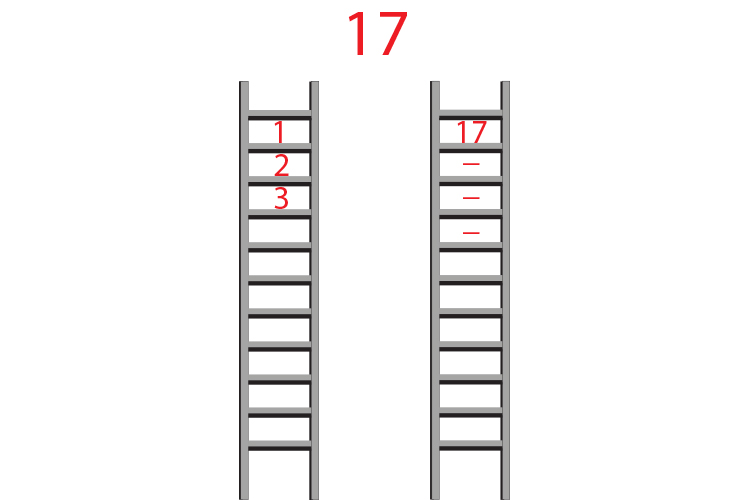There are no other combinations that work.

17 is a prime number.

So 17 is the prime factorisation.